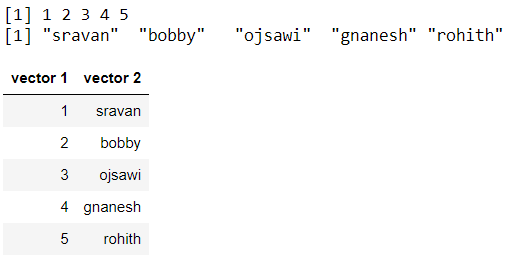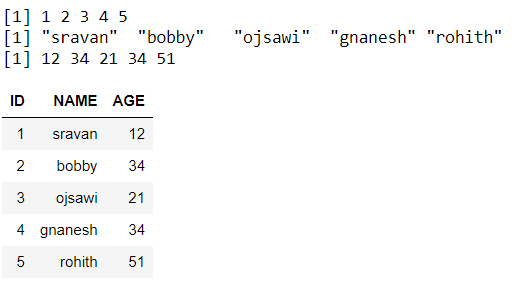Related Articles

# Set Column Names when Using cbind Function in R

• Last Updated : 23 Aug, 2021

In this article, we are going to see how to set the column names when using cbind() function in R Programming language.

Let’s create and combine two vectors for demonstration:

## R

 `# create two vectors with integer and string types``vector1 = ``c``(1,2,3,4,5)`` ` `vector2 = ``c``(``"sravan"``,``"bobby"``,``            ``"ojsawi"``,``"gnanesh"``,``            ``"rohith"``)`` ` `# display``print``(vector1)``print``(vector2)`` ` `# combine two vectors using cbind()``print``(``cbind``(vector1, vector2))`

Output:

``` 1 2 3 4 5
 "sravan"  "bobby"   "ojsawi"  "gnanesh" "rohith"
vector1 vector2
[1,] "1"     "sravan"
[2,] "2"     "bobby"
[3,] "3"     "ojsawi"
[4,] "4"     "gnanesh"
[5,] "5"     "rohith"```

## Method 1 : Set column names using colnames() function

colnames() function in R Language is used to set the names to columns of a matrix.

Syntax:
colnames(x) <- value

Parameters:
x: Matrix
value: Vector of names to be set

Example: R program to set the column names using colnames() function.

## R

 `# create two vectors with integer and string types``vector1 = ``c``(1,2,3,4,5)`` ` `vector2 = ``c``(``"sravan"``,``"bobby"``,``"ojsawi"``,``            ``"gnanesh"``,``"rohith"``)`` ` `# display``print``(vector1)``print``(vector2)`` ` `# combine two vectors using cbind()``combined = ``cbind``(vector1,vector2)`` ` `# Applying colnames``colnames``(combined) = ``c``(``"vector 1"``, ``"vector 2"``)    `` ` `# display``combined`

Output:## Method 2 : Set column names within cbind() function

Here, we have to give column names in the cbind() function itself.

Syntax: cbind(column_name = data1, column_name= data2,………,column_name=data n)

where

1. data is the input data to be combines
2. column_name is the new column name.

Example: R program to set the column name within cbind() function for three vectors

## R

 `# create three vectors with integer and string types``vector1 = ``c``(1,2,3,4,5)`` ` `vector2 = ``c``(``"sravan"``,``"bobby"``,``"ojsawi"``,``            ``"gnanesh"``,``"rohith"``)`` ` `vector3 = ``c``(12,34,21,34,51)`` ` `# display``print``(vector1)``print``(vector2)``print``(vector3)`` ` `# combine three vectors with in  cbind() function``combined = ``cbind``(``"ID"``=vector1,``                 ``"NAME"``=vector2,``"AGE"``=vector3)`` ` `# display``combined`

Output:My Personal Notes arrow_drop_up